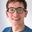# Mambu Banking Engine

## Interest calculated as percentage of loan amount

What

An interest calculation method using the total loan amount and a flat percentage, irrespective of the length of the loan. For instance:

Loan amount: \$1000

Interest percentage: 30%

Total interest: \$300

Why

Some organisations do not use interest rates, but rather calculate an amount for the whole loan based on the original loan amount. At present this can be worked around by manually calculating the overall interest then working backwards to reach the interest rate, or by using fees. It would naturally be more convenient and less cumbersome to have Mambu calculate the relevant amounts and generate the schedule on creation of the loan.

•James Anderson
• Nov 20 2020
•